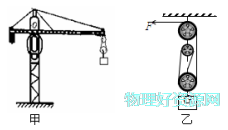# 如图是建筑工地上的塔式起重机示意图，它是通过电动机带动如图乙所示滑轮组起吊物料的。如果这个滑1）拉力的功率；

2）该滑轮组的动滑轮重；

3）该滑轮组的机械效率。

1条回答
• 解析：
1）绳端拉出的速度

v=s/t=nh/t=3×10m/10s

=3m/s

拉力的功率是
P=Fv=2.1×103N×3m/s

=6.3×103W

2）其动滑轮的重为

G=nF-G

=3×2.1×103N-6×103N

=300N

3

η=W有用/W=Gh/(FS)

=Gh/(Fnh)

=G/(nF)

=6×103N/(3×2.1×103N)

=95.24%

讨论(0)看不清?